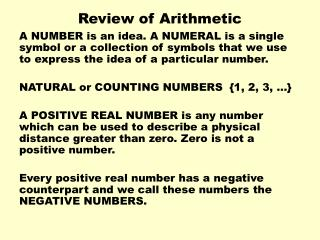DownloadDownload PresentationReview of Arithmetic

# Review of Arithmetic

Download Presentation## Review of Arithmetic

- - - - - - - - - - - - - - - - - - - - - - - - - - - E N D - - - - - - - - - - - - - - - - - - - - - - - - - - -
##### Presentation Transcript

1. Review of Arithmetic A NUMBER is an idea. A NUMERAL is a single symbol or a collection of symbols that we use to express the idea of a particular number. NATURAL or COUNTING NUMBERS {1, 2, 3, …} A POSITIVE REAL NUMBER is any number which can be used to describe a physical distance greater than zero. Zero is not a positive number. Every positive real number has a negative counterpart and we call these numbers the NEGATIVE NUMBERS.

2. NUMBER LINES A NUMBER LINE is a line which has been divided into units of equal length. WE choose a point on the line as our base point. This point is called the ORIGIN and we always associate the number ZERO with this point. When we place a dot on the number line to indicate the location of a number, we say that we have GRAPHED the number and the DOT is the GRAPH of the number. The number associated with the dot is the COORDINATE of the point we have graphed. One number is GREATER than another number if its graph lies to the RIGHT of the other number.

3. Multiplication and Division of Fractions Fractions are multiplied by multiplying numerators to get the new numerator and by multiplying denominators to get the new denominator. Ex.: 4/3 x 7/5 = 28/15 = 1 13/15 To divide fractions we invert the divisor and multiply i.e. multiply the first fraction by the reciprocal of the second fraction. Ex.: 4/3 ÷ 15/8 = 4/3 x 8/15 = 32/45 Always cancel first if possible.

4. Symbols of Equality & Inequality – Basic Operations ( Addition, Subtraction, Multiplication, Division) The equal sign, =, means that two quantities are equal i. e. 5 + 2 = 7 We use an equal sign with a slash through it to indicate that two quantities are not equal. We call two numbers we are adding the ADDENDS. The result is called the SUM. In subtraction the first number is called the MINUEND and the second number is called the SUBTRAHEND. The result of subtraction is call the DIFFERENCE.

5. Basic Operations continued In multiplication each of the numbers being multiplied is call a FACTOR. The result of multiplication is called a PRODUCT. If one number is being divided by another number the first number is called a DIVIDEND and the second number is called the DIVISOR. The result of division is call the QUOTIENT. Operations with decimal numbers, unit multipliers, and conversions of length. MEMORIZE THE CHART ON p. 21 On Line Tutorial for Unit Multipliers